Introduction to Atomic Structure: Various Models of Atom

# Introduction to Atomic Structure: Various Models of Atom - Notes | Study Physical Chemistry - Chemistry

 Table of contentsAtomic Structure: Discovery of Subatomic ParticlesDalton’s Theory of AtomsVarious Models of AtomImportant Atomic TermsBohr’s Atomic Model1 Crore+ students have signed up on EduRev. Have you?

Atomic Structure: Discovery of Subatomic Particles

Atomic structure refers to the structure of an atom comprising a nucleus (centre) in which protons (positively charged) and neutrons (neutral) are present. Negatively charged particles called electrons revolve around the centre of the nucleus.

• The history of atomic structure and quantum mechanics dates back to the times of Democritus, the man who first proposed that matter is composed of atoms.
• The study of the structure of an atom gives great insight into the entire class of chemical reactions, bonds, and their physical properties.
• The first scientific theory of atomic structure was proposed by John Dalton in the 1800s.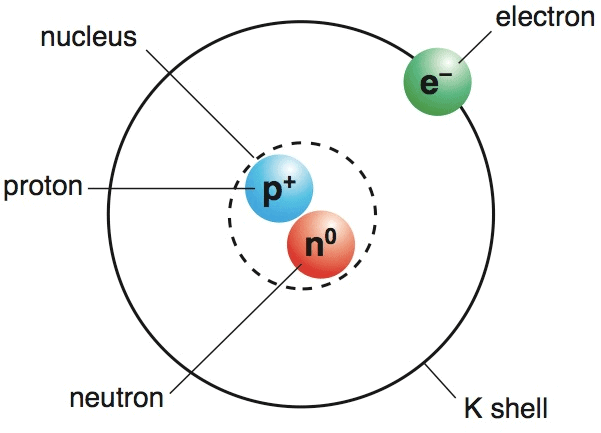Dalton’s Theory of Atoms

According to this theory the Atom is considered to be the hardest, densest, and smallest particle of matter that is indivisible. The atoms belonging to a particular element, are unique.

• The properties of elements differ because of the uniqueness of the atoms that belong to particular elements.
• This theory provides a satisfactory basis for the laws of chemical combination. The atom can neither be created nor be destroyed, i.e., it is indestructible.

Drawbacks of Dalton's Theory of Atoms

It fails to explain why atoms of different kinds should differ in mass and valency etc.

Sub-Atomic Particles

The discovery of various sub-atomic particles like electrons, protons, etc. during the late 19th century led to the idea that the atom was no longer an indivisible, small particle of matter.

Characteristics of the three fundamental particles are: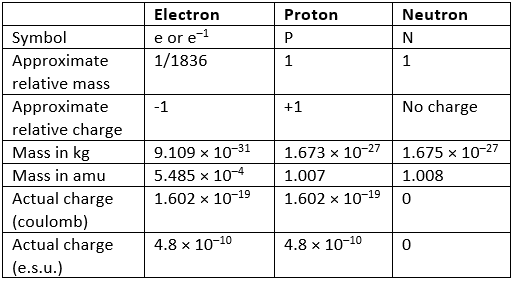Atomic Mass Unit: The atomic mass unit (amu) is 1/12 of the mass of an individual atom of 6C12, i.e., 1.660 × 10h – 27 kg. The neutron and proton have approximately equal masses of 1 amu and the electron is about 1836 times lighter. Its mass can sometimes be neglected as an approximation.

The electron and proton have an equal, but opposite, electric charges while the neutron is not charged.

Various Models of Atom

Thomson’s Model of Atom

Thomson assumed that an atom is a sphere of positive charges uniformly distributed, with the electrons scattered as points throughout the sphere.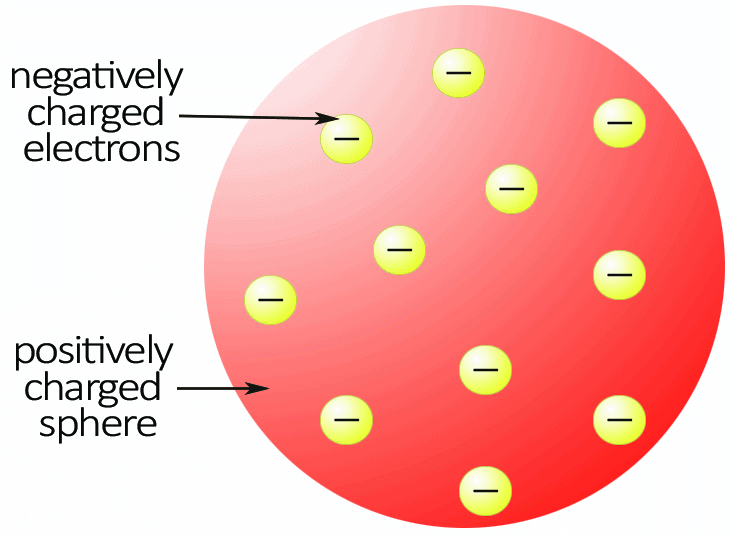• This was known as the "plum pudding model" at that time.
• However, this idea was dropped due to the success of the a-particle scattering experiments studied by Rutherford and Marsden.

Rutherford’s Model of Atom

Rutherford's model of the atom describes that the atom is composed of an atomic nucleus and electrons surrounding the nucleus. This model caused to reject the Thomson model of atom.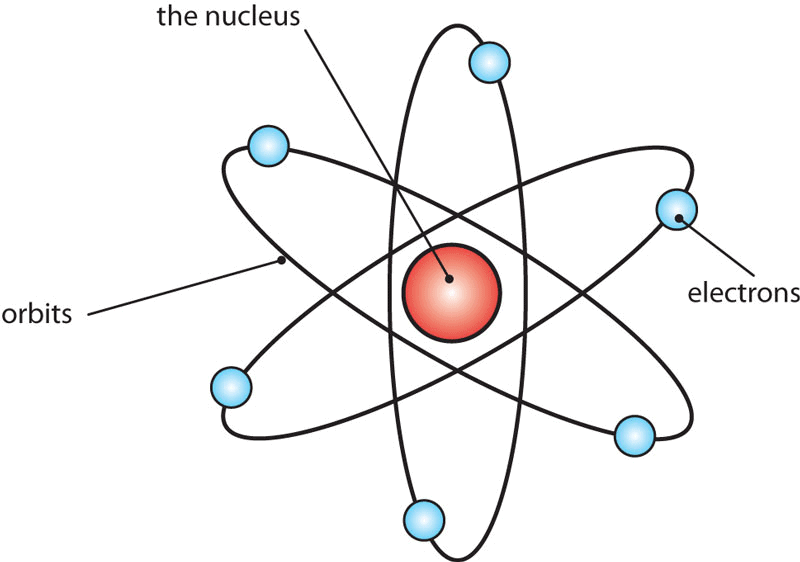• alpha-particle emitted by radioactive substances was shown to be dipositive Helium ions (He++) having a mass of 4 units and 2 units of positive charge.
• Rutherford allowed a narrow beam of α-particles to fall on a very thin gold foil of a thickness of the order of 4 × 10–4 cm and determined the subsequent path of these particles with the help of a zinc sulfide fluorescent screen.
• This zinc sulfide screen gives off a visible flash of light when struck by an α-particle, as ZnS has the remarkable property of converting the kinetic energy of particles into visible light.

Observation

• The majority of the α-particles pass straight through the gold strip with little or no deflection.
• Some particles are deflected from their path and diverge.
• Very few particles are deflected backwards through angles greater than 90°
• Some were ever scattered in the opposite direction at an angle of 180°

Conclusion

• The fact that most of the a-particles passed straight through the metal foil indicates that most part of the atom is empty.
• The fact that few alpha-particles are deflected at large angles indicates the presence of a heave positively charged body i.e., for such large deflections to occur a-particles must have some closer to or collide with a massive positively charged body, and he named its nucleus.
• The fact that one in 20,000 have deflected at 180° backward indicates that the volume occupied by this heavy positively charged body is very small in comparison to the total volume of the atom

Drawbacks of Rutherford’s Atomic Model

• Position of electrons: The exact positions of the electrons from the nucleus are not mentioned.
• Stability of the atom: Neils Bohr pointed out that Rutherford’s atom should be highly unstable. According to the law of electrodynamics, the electron should, therefore, continuously emit radiation and lose energy. As a result of this, a moving electron will come closer and closer to the nucleus and after passing through a spiral path, it should ultimately fall into the nucleus.
• It was calculated that electrons should fall into the nucleus in less than 10–8 sec. But it is known that electrons keep moving outside the nucleus.
• To solve this problem Neils Bohr proposed an improved form of Rutherford’s atomic model.

Before going into the details of the Neils Bohr model we would like to introduce you to some important atomic terms.

Important Atomic Terms
Dark Line in Absorption Spectrum

If the atom gains energy the electron passes from a lower energy level to a higher energy level, energy is absorbed which means a specific wavelength is absorbed. Consequently, a dark line will appear in the spectrum. This dark line constitutes the absorption spectrum.

Hydrogen Atom

If an electric discharge is passed through hydrogen gas taken in a discharge tube under low pressure, and the emitted radiation is analyzed with the help of a spectrograph, it is found to consist of a series of sharp lines in the UV, visible, and IR regions. This series of lines is known as a line or atomic spectrum of hydrogen. The lines in the visible region can be directly seen on the photographic film.

Each line of the spectrum corresponds to light of a definite wavelength. The entire spectrum consists of six series of lines each, known after their discoverer as the Balmer, Paschen, Lyman, Brackett, Pfund, and Humphrey series. The wavelength of all these series can be expressed by a single formula.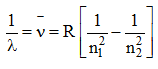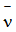= wave number

l = wave length

R = Rydberg constant (109678 cm–1)

n1 and n have integral values as follows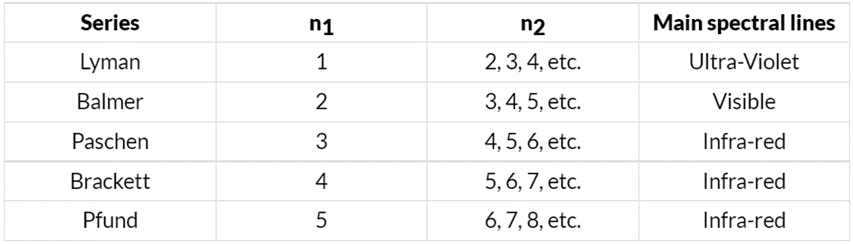Types of Emission Spectra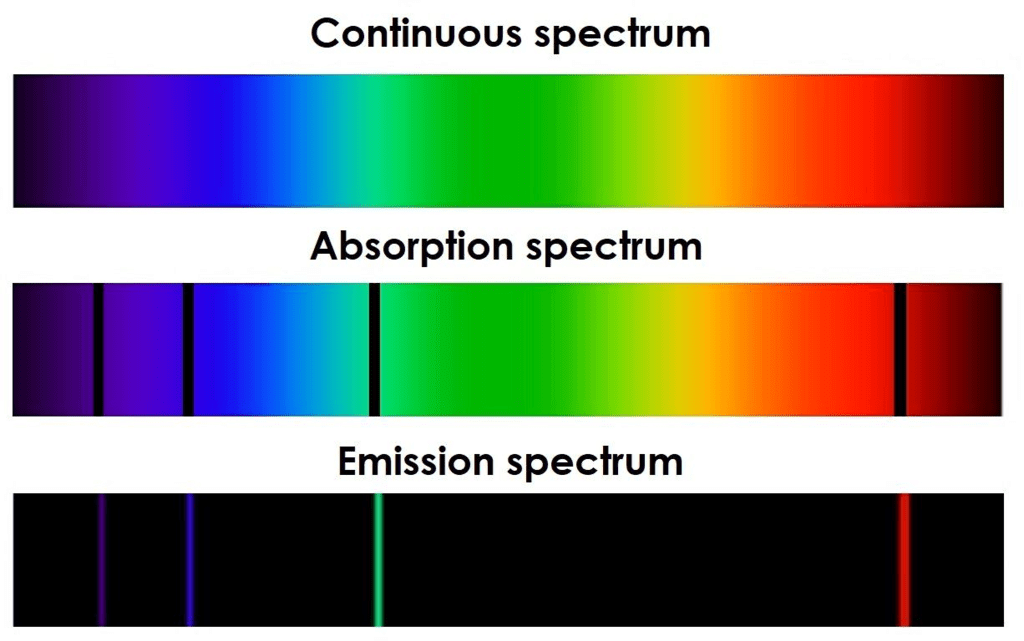• Continuous Spectra: When white light from any source such As sun or bulb is analyzed by passing through a prism, it splits up into seven different wide bands of colour from violet to red (like a rainbow). These colours are also continuous that each of them merges into the next. Hence the spectrum is called a continuous spectrum.
• Line Spectra: When the electric discharge is passed through a gas at low pressure light is emitted. If this light is resolved by a spectroscope, it is found that some isolated coloured lines are obtained on a photographic plate separated from each other by dark spaces. This spectrum is called the line spectrum. Each line in the spectrum corresponds to a particular wavelength. Each element gives its own characteristic spectrum.

Planck’s Quantum Theory

When a black body is heated, it emits thermal radiation of different wavelengths or frequencies. To explain these radiations, Max Planck put forward a theory known as Planck’s theory. The main points of quantum theory are:

• Substances radiate or absorb energy discontinuously in the form of small packets or bundles of energy.
• The smallest packet of energy is called quantum. In the case of light, the quantum is known as a photon.
• The energy of a quantum is directly proportional to the frequency of the radiation. E∝ ν ( or E= hv where v is the frequency of radiation and h is Planck’s constant having the value 6.626 × 10–27 erg-sec or 6.626 × 10–34 J-sec.
• A body can radiate or absorb energy in whole-number multiple of a quantum hv, 2hv, 3hv, nhν where n is the positive integer. Nelis Bohr used this theory to explain the structure of atoms.

Bohr’s Atomic Model

Bohr developed a model for hydrogen and hydrogen-like atoms one-electron species (hydrogenic species). He applied quantum theory in considering the energy of an electron bound to the nucleus.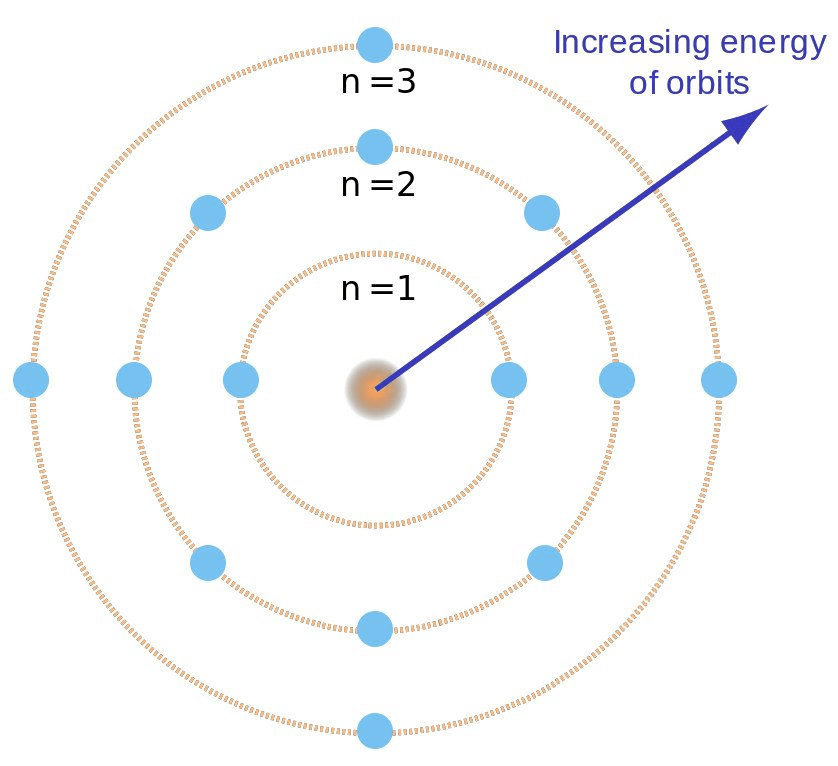Important Postulates

• An atom consists of a dense nucleus situated at the center with the electron revolving around it in circular orbitals without emitting any energy. The force of attraction between the nucleus and an electron is equal to the centrifugal force of the moving electron.
• Of the finite number of circular orbits possible around the nucleus, the electron can revolve only in those orbits whose angular momentum (mvr) is an integral multiple of factor h/2p.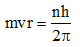Where m = mass of the electron
v = velocity of the electron
n = orbit number on which electron is present
r = radius of the orbit
• As long as an electron is revolving in an orbit it neither loses nor gains energy. Hence these orbits are called stationary states. Each stationary state is associated with a definite amount of energy and it is also known as energy levels. The greater the distance of the energy level from the nucleus, the more is the energy associated with it. The different energy levels are numbered as 1, 2, 3, 4 (from nucleus onwards) or K, L, M, N etc.
• Ordinarily, an electron continues to move in a particular stationary state without losing energy. Such a stable state of the atom is called a ground state or normal state.
• If energy is supplied to an electron, it may jump (excite) instantaneously from lower energy (say 1) to higher energy level (say 2, 3, 4, etc) by absorbing one quantum of energy. This new state of the electron is called an excited state.
• The quantum of energy absorbed is equal to the difference in energies of the two concerned levels. Since the excited state is less stable, the atom will lose its energy and come back to the ground state.
• Energy absorbed or released in an electron jump, (ΔE) is given by
ΔE = E2 – E1 = hv
Where E2 and E1 are the energies of the electron in the first and second energy levels, and v is the frequency of radiation absorbed or emitted.

Merits of Bohr’s theory

• The experimental value of radii and energies in a hydrogen atom is in good agreement with that calculated on the basis of Bohr’s theory.
• Bohr’s concept of the stationary state of electrons explains the emission and absorption spectra of hydrogen-like atoms.
• The experimental values of the spectral lines of the hydrogen spectrum are in close agreement with the calculated by Bohr’s theory.

Limitations of Bohr’s Theory

• It does not explain the spectra of atoms or ions having more than one electron.
• Bohr’s atomic model failed to account for the effect of magnetic field (Zeeman effect) or electric field (Stark effect) on the spectra of atoms or ions. It was observed that when the source of a spectrum is placed in a strong magnetic or electric field, each spectral line further splits into a number of lines. This observation could not be explained on the basis of Bohr’s model.
• De-Broglie suggested that electrons like light have a dual character. It has particle and wave characters. Bohr treated the electron only as a particle.
• Another objection to Bohr’s theory came from Heisenberg’s Uncertainty Principle. According to this principle, “it is impossible to determine simultaneously the exact possible and momentum of a small moving particle like an electron”. The postulate of Bohr, that electrons revolve in well-defined orbits around the nucleus with well-defined velocities is thus not attainable.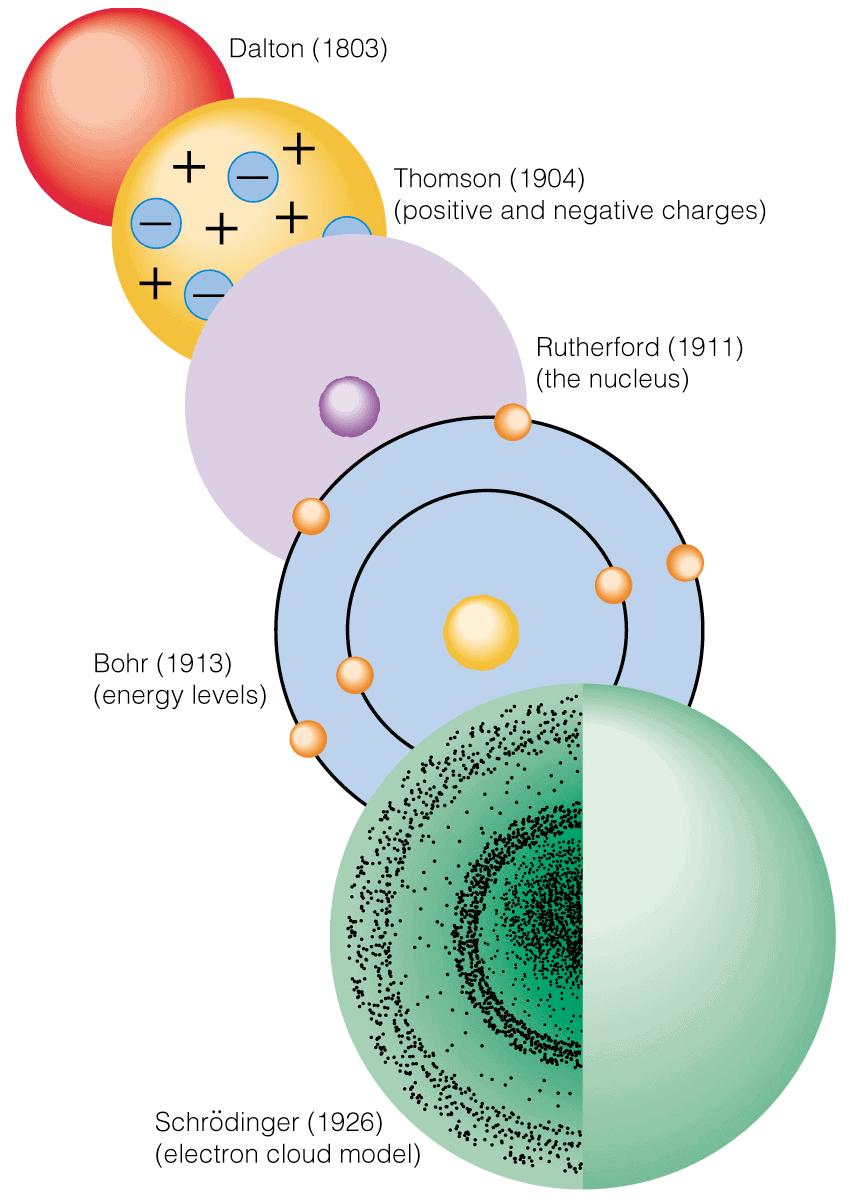Results of Bohr's Theory

Radius and Energy levels of the hydrogen atom

Consider an electron of mass ‘m’ and charge ‘e’ revolving around a nucleus of charge Ze (where Z = atomic number and e is the charge of the proton) with a tangential velocity v.r is the radius of the orbit in which electron is revolving.
By Coulomb’s Law, the electrostatic force of attraction between the moving electron and nucleus is

Coulombic force =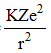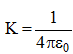(where ε0 is the permittivity of free space)

K = 9 × 109 Nm2C–2

In C.G.S units, value of K = 1 dyne cm2 (esu)–2

The centrifugal force acting on the electron is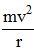Since the electrostatic force balance the centrifugal force, for the stable electron orbit.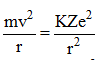...............(i)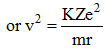...............(ii)

According to Bohr’s postulate of angular momentum quantization, we have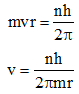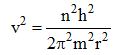............ (iii)

Equating (ii) and (iii)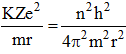Solving for r we get r =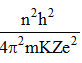Where n = 1, 2, 3, ………….∞

Hence only certain orbits whose radii are given by the above equation are available for the electron. The greater the value of n, i.e., the farther the level from the nucleus the greater is the radius.

The radius of the smallest orbit (n = 1) for the hydrogen atom (Z = 1) is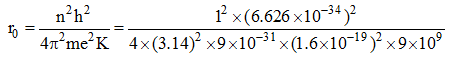r0 = 0.529 Å

The radius of nth orbit for an atom with atomic number Z is simply written as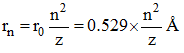Illustration: Calculate the ratio of the radius of Li+2 ion in 3rd energy level to that of He+ ion in 2nd energy level:

Solution: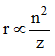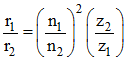n1 = 3

n2 = 3

z1 = 3                                       (for Li2+)

z2 = 2                                       (for He+)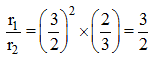The Energy Level of Hydrogen atom:

• The total energy, E of the electron is the sum of kinetic energy and potential energy. The kinetic energy of the electron = 1/2 mv2
• Potential energy =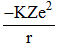• Total energy =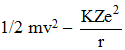………..(4)
From equation (1) we know that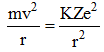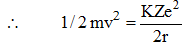Substituting this in equation (4)
Total energy (e)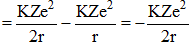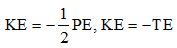Substituting for r, gives us
E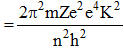where n 1, 2, 3.........
This expression shows that only certain energies are allowed to the electron. Since this energy expression consists of so many fundamental constants, we are giving you the following, simplified expressions.
E =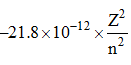erg per Atom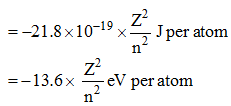(1 eV = 3.83 × 10–23 Kcal)
(1 eV = 1.602 × 10–12 erg)
(1 eV = 1.602 × 10–19 J)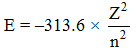kcal/mole (1cal = 4.18 J)
The energies are negative since the energy of the electron in the atom is less than the energy of a free electron (i.e., the electron is at an infinite distance from the nucleus) which is taken as zero. The lowest energy level of the atom corresponds to n = 1, and as the quantum number increases, E becomes less negative.
When n = ∞, E = 0 which corresponds to an ionized atom i.e., the electron and nucleus are infinitely separated.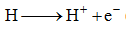• Velocity of Electron:
We know that mvr =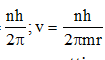By substituting for r we are getting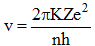Where excepting n and z all are constant, v =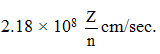Further application of Bohr’s work was made, to other one-electron species (Hydrogenic ion) such as He+ and Li2+. In each case of this kind, Bohr’s prediction of the spectrum was correct.
The document Introduction to Atomic Structure: Various Models of Atom - Notes | Study Physical Chemistry - Chemistry is a part of the Chemistry Course Physical Chemistry.
All you need of Chemistry at this link: Chemistry

## Physical Chemistry

85 videos|131 docs|67 tests
 Use Code STAYHOME200 and get INR 200 additional OFF

## Physical Chemistry

85 videos|131 docs|67 tests

Track your progress, build streaks, highlight & save important lessons and more!

,

,

,

,

,

,

,

,

,

,

,

,

,

,

,

,

,

,

,

,

,

;